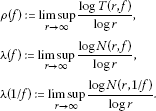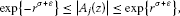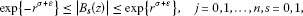Theory and Modern Applications

# Results on meromorphic solutions of linear difference equations

## Abstract

In this paper, we investigate meromorphic solutions of linear difference equations and prove a number of results. We give estimates for the growth of meromorphic solutions under some special cases and provide some examples to show that the answer to a question of Laine and Yang is not always positive. The zeros, poles and fixed points of finite order solutions are also studied.

MSC:39A13, 39A22, 30D35.

## 1 Introduction and results

In this paper, a meromorphic function means meromorphic in the complex plane, and we assume the reader is familiar with the basic notions of Nevanlinna theory (see, e.g., ). We use the notations $\rho \left(f\right)$, $\lambda \left(f\right)$, $\lambda \left(1/f\right)$ to denote the order of growth of f, the exponent of convergence of the poles of f and the exponent of convergence of the zeros of f, respectively, and we define them as follows:Thirty years ago, some results on the existence of meromorphic solutions for certain difference equations were proved by Shimomura  and Yanagihara .

Recently, numbers of papers (see, e.g., ) are devoted to considering the complex difference equations and difference analogues of Nevanlinna theory. For the growth of meromorphic solutions of difference equations, Chiang and Feng [8, 9] considered the polynomial coefficients case and got

Theorem A [8, 9]

Let ${A}_{0}\left(z\right),{A}_{1}\left(z\right),\dots ,{A}_{n}\left(z\right)$ be polynomials such that there exists an integer l, $0\le l\le n$, such that

$deg\left({A}_{l}\right)>\underset{0\le j\le n,j\ne l}{max}\left\{deg\left({A}_{j}\right)\right\}.$

Suppose that $f\left(z\right)$ is a meromorphic solution to

${A}_{n}\left(z\right)f\left(z+n\right)+\cdots +{A}_{1}\left(z\right)f\left(z+1\right)+{A}_{0}\left(z\right)f\left(z\right)=0,$
(1.1)

then we have $\rho \left(f\right)\ge 1$.

The following result shows that the polynomial coefficients in Theorem A can be extended to rational functions.

Theorem 1.1 Let ${A}_{0}\left(z\right),{A}_{1}\left(z\right),\dots ,{A}_{n}\left(z\right)$ be rational functions having no common zeros or poles. For $j=0,\dots ,n$, set ${A}_{j}\left(z\right)={p}_{j}\left(z\right)/{q}_{j}\left(z\right)$, where ${p}_{j}\left(z\right)$, ${q}_{j}\left(z\right)$ are irreducible polynomials. If there exists an integer l, $0\le l\le n$, such that

$deg\left({p}_{l}\right)-deg\left({q}_{l}\right)>\underset{0\le j\le n,j\ne l}{max}\left\{deg\left({p}_{j}\right)-deg\left({q}_{j}\right)\right\},$

then, for any meromorphic solution $f\left(z\right)$ to (1.1), we have $\rho \left(f\right)\ge 1$.

Remark Set ${B}_{j}={p}_{j}{\prod }_{k=0}^{n}{q}_{k}/{q}_{j}$, then we see that ${B}_{0}\left(z\right),{B}_{1}\left(z\right),\dots ,{B}_{n}\left(z\right)$ are all polynomials and

$deg\left({B}_{l}\right)>\underset{0\le j\le n,j\ne l}{max}\left\{deg\left({B}_{j}\right)\right\}.$

And hence Theorem 1.1 follows from Theorem A. We omit the details of its proof.

For the case that some coefficients are transcendental meromorphic functions, the following two results were proved by Chaing and Feng  and Laine and Yang , respectively.

Theorem B 

Let ${A}_{0}\left(z\right),{A}_{1}\left(z\right),\dots ,{A}_{n}\left(z\right)$ be entire functions such that there exists an integer l, $0\le l\le n$, such that

$\rho \left({A}_{l}\right)>\underset{0\le j\le n,j\ne l}{max}\rho \left({A}_{j}\right).$

If $f\left(z\right)$ is a meromorphic solution to (1.1), then $\rho \left(f\right)\ge \rho \left({A}_{l}\right)+1$.

Theorem C 

Let ${A}_{0}\left(z\right),{A}_{1}\left(z\right),\dots ,{A}_{n}\left(z\right)$ be entire functions of finite order such that among those coefficients having the maximal order $\rho :={max}_{0\le j\le n}\rho \left({A}_{j}\right)$, exactly one has its type strictly greater than the others. Then, for any meromorphic solution $f\left(z\right)$ to (1.1), we have $\rho \left(f\right)\ge \rho +1$.

In Theorems B and C, there is always some dominating coefficient ${A}_{l}$ such that $\rho \left({A}_{l}\right)>0$. A natural question is what happens if the dominating coefficient ${A}_{l}$ is of order zero? Another question raised by Laine and Yang in  is whether all meromorphic solutions $f\left(z\right)$ of (1.1) satisfy $\rho \left(f\right)\ge {max}_{0\le j\le n}\rho \left({A}_{j}\right)+1$, even if there is no dominating coefficient. For the first question, we get the following result.

Theorem 1.2 Let ${A}_{0}\left(z\right),{A}_{1}\left(z\right),\dots ,{A}_{n}\left(z\right)$ be meromorphic functions such that there exists an integer l, $0\le l\le n$, such that ${A}_{l}\left(z\right)$ is a transcendental entire function, while ${A}_{j}\left(z\right)$, $j\ne l$, are all rational functions. If $f\left(z\right)$ is a meromorphic solution to (1.1), then $\rho \left(f\right)\ge \rho \left({A}_{l}\right)+1$.

Considering Laine and Yang’s question, we get the following example which indicates that the answer to their question is not always positive.

Example For a given positive integer k, $f\left(z\right)={e}^{z}$ is an entire solution of the equation

$\left({e}^{{z}^{k}-2}+1\right)f\left(z+2\right)-{e}^{{z}^{k}-1}f\left(z+1\right)-{e}^{2}f\left(z\right)=0.$

In this example, the relationship between $\rho \left(f\right)$ and ${max}_{0\le j\le n}\rho \left({A}_{j}\right)+1=k+1$ exactly depends on k.

However, the answer may be positive in some special case. In fact, we prove the following results, in which there is still some coefficient dominating in some angle.

Theorem 1.3 Let k be a positive integer, p be a nonzero real number and $f\left(z\right)$ be a nonconstant meromorphic solution of the difference equation

${A}_{n}f\left(z+n\right)+\cdots +{A}_{2}f\left(z+2\right)+\left({A}_{1}{e}^{-p{z}^{k}}+{B}_{1}\right)f\left(z+1\right)+\left({A}_{0}{e}^{p{z}^{k}}+{B}_{0}\right)f\left(z\right)=0,$
(1.2)

where ${A}_{0},{A}_{1},\dots ,{A}_{n},{B}_{0},{B}_{1}$ are all entire functions such that ${A}_{0}{A}_{1}\ne 0$ and $max\left\{\rho \left({B}_{0}\right),\rho \left({B}_{1}\right),\rho \left({A}_{j}\right):0\le j\le n\right\}=\sigma . Then we have $\rho \left(f\right)\ge k+1$.

Theorem 1.4 Under exactly one of assumptions for the coefficients of (1.1) in Theorems  A-C and Theorems 1.1 and 1.2, if $f\left(z\right)$ is a finite order meromorphic solution to (1.1), then $\lambda \left(f-z\right)=\rho \left(f\right)$. What is more, either $\rho +1\le \rho \left(f\right)\le max\left\{\lambda \left(f\right),\lambda \left(1/f\right)\right\}+1$ or $\rho \left(f\right)=\rho +1>max\left\{\lambda \left(f\right),\lambda \left(1/f\right)\right\}+1$, where $\rho :={max}_{0\le j\le n}\rho \left({A}_{j}\right)$.

Theorem 1.5 Under the assumption for the coefficients of (1.2) in Theorem  1.3, if $f\left(z\right)$ is a finite order meromorphic solution to (1.2), then $\lambda \left(f-z\right)=\rho \left(f\right)$. What is more, either $k+1\le \rho \left(f\right)\le max\left\{\lambda \left(f\right),\lambda \left(1/f\right)\right\}+1$ or $\rho \left(f\right)=k+1>max\left\{\lambda \left(f\right),\lambda \left(1/f\right)\right\}+1$.

The following examples show the sharpness of the estimates in Theorems 1.4 and 1.5.

Examples (1) The gamma function $\mathrm{\Gamma }\left(z\right)$ is a meromorphic solution to the equation

$f\left(z+1\right)-zf\left(z\right)=0,$

which satisfies the assumptions in Theorem A and Theorem 1.1. We see that $\lambda \left(\mathrm{\Gamma }-z\right)=\rho \left(\mathrm{\Gamma }\right)$ and $1=\rho \left(\mathrm{\Gamma }\right)<\lambda \left(1/\mathrm{\Gamma }\right)+1$ .

(2) ${f}_{1}\left(z\right)=z{e}^{{z}^{2}}$ and ${f}_{2}\left(z\right)=z{e}^{{z}^{2}}sin\left(2\pi z\right)$ are entire solutions to the equation

$z{e}^{-2z}f\left(z+1\right)-e\left(z+1\right)f\left(z\right)=0,$

which satisfies the assumptions in Theorems B and C and Theorem 1.2. We have $\lambda \left({f}_{1}-z\right)=\rho \left({f}_{1}\right)$, $\lambda \left({f}_{2}-z\right)=\rho \left({f}_{2}\right)$ and $\rho \left({f}_{1}\right)=2>\lambda \left({f}_{1}\right)+1$, $\rho \left({f}_{2}\right)=2=\lambda \left({f}_{2}\right)+1$.

(3) ${f}_{1}\left(z\right)={e}^{{z}^{2}}sin2\pi z$ and ${f}_{2}\left(z\right)={e}^{{z}^{2}}$ are entire solutions of the equation

${e}^{-z}f\left(z+1\right)-e\cdot {e}^{z}f\left(z\right)=0,$

which satisfies the assumptions in Theorem 1.3. We have $\lambda \left({f}_{1}-z\right)=\rho \left({f}_{1}\right)$, $\lambda \left({f}_{2}-z\right)=\rho \left({f}_{2}\right)$ and $\rho \left({f}_{1}\right)=2=1+\lambda \left({f}_{1}\right)$ and $\rho \left({f}_{2}\right)=2>\lambda \left({f}_{2}\right)+1$.

## 2 Proof of Theorem 1.2

We first recall a key lemma used to prove Theorems A and B and the pointwise estimates for difference quotient which are counterparts to Gundersen’s logarithmic derivative estimates  (see , Corollary 2.6, Theorem 8.3).

Lemma 2.1 

Let $f\left(z\right)$ be a meromorphic function of finite order ρ, ε be a positive constant, ${\eta }_{1}$ and ${\eta }_{2}$ be two distinct nonzero complex constants. Then

$m\left(r,\frac{f\left(z+{\eta }_{1}\right)}{f\left(z+{\eta }_{2}\right)}\right)=O\left({r}^{\rho -1+\epsilon }\right),$

and there exists a subset $E\subset \left(1,+\mathrm{\infty }\right)$ of finite logarithmic measure such that, for all z satisfying $|z|=r\notin \left[0,1\right]\cup E$, and as $r\to \mathrm{\infty }$ sufficiently large,

$exp\left\{-{r}^{\rho -1+\epsilon }\right\}\le |\frac{f\left(z+{\eta }_{1}\right)}{f\left(z+{\eta }_{2}\right)}|\le exp\left\{{r}^{\rho -1+\epsilon }\right\}.$

Proof of Theorem 1.2 If $\rho \left({A}_{l}\right)>0$, the assertion follows from Theorem B. We next consider the case that $\rho \left({A}_{l}\right)=0$.

Assume that $\rho \left(f\right)<1$, then by Lemma 2.1 (or , Lemma 3.3), it is easy to deduce that for $j\ne l$, there exists a subset $E\subset \left(1,+\mathrm{\infty }\right)$ of finite logarithmic measure such that, for all $z=r{e}^{i\theta }$, $\theta \in \left[0,2\pi \right)$, $|z|=r\notin \left[0,1\right]\cup E$, and as $r\to \mathrm{\infty }$,

$\frac{f\left(z+j\right)}{f\left(z+l\right)}\to 1.$
(2.1)

For $j\ne l$, set ${A}_{j}\left(z\right)={p}_{j}\left(z\right)/{q}_{j}\left(z\right)$, where ${p}_{j}\left(z\right)$, ${q}_{j}\left(z\right)$ are irreducible polynomials. Denote $d=max\left\{0,deg\left({p}_{j}\right)-deg\left({q}_{j}\right):0\le j\le n,j\ne l\right\}$. Since ${A}_{l}$ is a transcendental entire function, for sufficiently large r, we have

$M\left(r,{A}_{l}\right)>{r}^{d+2}.$
(2.2)

Now we choose a sequence ${z}_{k}={r}_{k}{e}^{i{\theta }_{k}}$, ${\theta }_{k}\in \left[0,2\pi \right)$, $|{z}_{k}|={r}_{k}\notin \left[0,1\right]\cup E$ such that $|{A}_{l}\left({z}_{k}\right)|=M\left({r}_{k},{A}_{l}\right)$, ${r}_{k}\to \mathrm{\infty }$ as $k\to \mathrm{\infty }$. Combining (1.1), (2.1) and (2.2), we get

${r}_{k}^{d+2}

a contradiction. Our proof is thus finished. □

## 3 Proof of Theorem 1.3

Our tools to prove Theorem 1.3 include the following Lemma 3.1 in which the upper bound is given by Gundersen  while the lower bound by Chen .

Lemma 3.1 [17, 18]

Let $f\left(z\right)$ be a meromorphic function with finite order ρ. Then, for any given $\epsilon >0$, there exists a set $E\subset \left(1,+\mathrm{\infty }\right)$ of finite linear measure such that, for all z satisfying $|z|=r\notin \left[0,1\right]\cup E$ and r sufficiently large,

$exp\left\{-{r}^{\rho +\epsilon }\right\}\le |f\left(z\right)|\le exp\left\{{r}^{\rho +\epsilon }\right\}.$

Proof of Theorem 1.3 Without loss of generality, we assume that $p=1$. Suppose that (1.2) admits a nontrivial entire solution $f\left(z\right)$ such that $\rho \left(f\right)=\rho . Then by Lemma 2.1, for any given ε such that $0<2\epsilon , we have

$exp\left\{-{r}^{\rho -1+\epsilon }\right\}\le |\frac{f\left(z+j\right)}{f\left(z\right)}|\le exp\left\{{r}^{\rho -1+\epsilon }\right\},\phantom{\rule{1em}{0ex}}j=1,\dots ,n,$
(3.1)

for all r outside of a possible exceptional set ${E}_{1}$ with finite logarithmic measure.

Applying Lemma 3.1, we have(3.2)(3.3)

for all r outside of a possible exceptional set ${E}_{2}$ with finite linear measure.

Choose an infinite positive real sequence ${z}_{t}={r}_{t}\notin {E}_{1}\cup {E}_{2}$ such that ${r}_{t}\to \mathrm{\infty }$ as $t\to \mathrm{\infty }$, and we get from (1.2), (3.1)-(3.3) that

$\begin{array}{rcl}exp\left\{{r}_{t}^{k}\right\}& =& |-{e}^{{z}_{t}^{k}}|\\ =& |\sum _{j=2}^{n}\frac{{A}_{j}\left({z}_{t}\right)}{{A}_{0}\left({z}_{t}\right)}\frac{f\left({z}_{t}+j\right)}{f\left({z}_{t}\right)}+\frac{{A}_{1}\left({z}_{t}\right){e}^{-{z}_{t}^{k}}+{B}_{1}\left({z}_{t}\right)}{{A}_{0}\left({z}_{t}\right)}\frac{f\left({z}_{t}+1\right)}{f\left({z}_{t}\right)}+\frac{{B}_{0}\left({z}_{t}\right)}{{A}_{0}\left({z}_{t}\right)}|\\ <& \left(n+2\right)exp\left\{2{r}_{t}^{\sigma +\epsilon }+{r}_{t}^{\rho -1+\epsilon }\right\},\end{array}$

a contradiction. And hence we have $\rho \left(f\right)\ge k+1$. □

## 4 Proofs of Theorems 1.4 and 1.5

Lemma 4.1 

Let ${f}_{j}\left(z\right)$ ($j=1,2,\dots ,n$, $n\ge 2$) be meromorphic functions and ${g}_{j}\left(z\right)$ ($j=1,2,\dots ,n$, $n\ge 2$) be entire functions such that

1. 1.

${\sum }_{j=1}^{n}{f}_{j}\left(z\right){e}^{{g}_{j}\left(z\right)}\equiv 0$,

2. 2.

${g}_{j}\left(z\right)-{g}_{k}\left(z\right)$ are not constant functions for $1\le j.

3. 3.

$T\left(r,{f}_{j}\right)=o\left(T\left(r,{e}^{{g}_{h}-{g}_{k}}\right)\right)$ ($r\to \mathrm{\infty }$, $r\notin E$), where E is an exceptional set of finite linear measure, $1\le j\le n$ and $1\le h.

Then ${f}_{j}\left(z\right)\equiv 0$ ($j=1,2,\dots ,n$).

Proofs of Theorems 1.4 and 1.5 In fact, we only give the proof of Theorem 1.5 since the proof of Theorem 1.4 is similar.

Suppose that $f\left(z\right)$ is a nonconstant meromorphic solution of (1.2) such that $\rho \left(f\right)<\mathrm{\infty }$. We firstly prove that $\lambda \left(f-z\right)=\rho \left(f\right)$. Submitting $f\left(z\right)=g\left(z\right)+z$ into (1.2), we get

${A}_{n}g\left(z+n\right)+\cdots +{A}_{2}g\left(z+2\right)+\left({A}_{1}{e}^{-p{z}^{k}}+{B}_{1}\right)g\left(z+1\right)+\left({A}_{0}{e}^{p{z}^{k}}+{B}_{0}\right)g\left(z\right)=D\left(z\right),$

where

$D\left(z\right)=-z\left\{{A}_{n}\left(z\right)+\cdots +{A}_{2}\left(z\right)+\left({A}_{1}\left(z\right){e}^{-p{z}^{k}}+{B}_{1}\left(z\right)\right)+\left({A}_{0}\left(z\right){e}^{p{z}^{k}}+{B}_{0}\left(z\right)\right)\right\}\not\equiv 0.$

Obviously, we have $\rho \left(D\right)\le k$.

Now, for any given $\epsilon >0$, applying Lemma 2.1, we can deduce that

$\begin{array}{rcl}m\left(r,\frac{1}{g}\right)& =& m\left(r,\frac{{A}_{n}g\left(z+n\right)+\cdots +\left({A}_{1}{e}^{-p{z}^{k}}+{B}_{1}\right)g\left(z+1\right)}{D\left(z\right)g\left(z\right)}+{A}_{0}{e}^{p{z}^{k}}+{B}_{0}\right)\\ \le & \sum _{j=1}^{n}m\left(r,\frac{g\left(z+j\right)}{g\left(z\right)}\right)+\sum _{j=0}^{n}T\left(r,{A}_{j}\right)+T\left(r,{B}_{0}\right)+T\left(r,{B}_{1}\right)\\ +T\left(r,{e}^{-p{z}^{k}}\right)+T\left(r,{e}^{p{z}^{k}}\right)+T\left(r,D\right)+O\left(logr\right)\\ =& O\left({r}^{\rho -1+\epsilon }\right)=S\left(r,g\right).\end{array}$

This implies that

$N\left(r,\frac{1}{f-z}\right)=N\left(r,\frac{1}{g}\right)=T\left(r,g\right)+S\left(r,g\right)=T\left(r,f\right)+S\left(r,f\right).$

Then $\lambda \left(f-z\right)=\rho \left(f\right)$ follows.

Next, we assert that either $k+1\le \rho \left(f\right)\le max\left\{\lambda \left(f\right),\lambda \left(1/f\right)\right\}+1$ or $\rho \left(f\right)=k+1$. If the assertion does not hold, we have $max\left\{k,\lambda \left(f\right),\lambda \left(1/f\right)\right\}+1<\rho \left(f\right)<\mathrm{\infty }$.

Assume that $z=0$ is a zero (or a pole) of $f\left(z\right)$ of order m. Applying the Hadamard factorization of a meromorphic function, we write $f\left(z\right)$ as follows:

$f\left(z\right)={z}^{m}\frac{{P}_{1}\left(z\right)}{{P}_{2}\left(z\right)}{e}^{Q\left(z\right)},$

where ${P}_{1}\left(z\right)$, ${P}_{2}\left(z\right)$ are entire functions such that $\rho \left({P}_{1}\right)=\lambda \left(f\right)$, $\rho \left({P}_{1}\right)=\lambda \left(1/f\right)$ and $Q\left(z\right)$ is a polynomial such that $degQ\left(z\right)=q>max\left\{k,\lambda \left(f\right),\lambda \left(1/f\right)\right\}+1$.

Now, we obtain from (1.2) that

$\sum _{j=1}{h}_{j}\left(z\right){e}^{Q\left(z+j-1\right)}=0,$
(4.1)

where

${h}_{1}=\left({A}_{0}{e}^{{z}^{k}}+{B}_{0}\right){z}^{m}\frac{{P}_{1}\left(z\right)}{{P}_{2}\left(z\right)},\phantom{\rule{2em}{0ex}}{h}_{2}=\left({A}_{1}{e}^{-{z}^{k}}+{B}_{1}\right){\left(z+1\right)}^{m}\frac{{P}_{1}\left(z+1\right)}{{P}_{2}\left(z+1\right)}$

and

${h}_{j}={\left(z+j-1\right)}^{m}\frac{{P}_{1}\left(z+j-1\right)}{{P}_{2}\left(z+j-1\right)}$

for $3\le j\le n+1$. Notice that $deg\left(Q\left(z+h\right)-Q\left(z+k\right)\right)=q-1>\rho \left({h}_{j}\right)$ for $1\le h and $0\le j\le n$. Thus, Lemma 4.1 is valid for (4.1) and hence we get that ${h}_{j}\left(z\right)\equiv 0$ for $j=0,1,\dots ,n$, a contradiction to our assumption. This completes our proof. □

## References

1. Hayman WK: Meromorphic Functions. Clarendon, Oxford; 1964.

2. Laine I: Nevanlinna Theory and Complex Differential Equations. de Gruyter, Berlin; 1993.

3. Yang CC, Yi HX Math. Appl. 557. In The Uniqueness Theory of Meromorphic Functions. Kluwer Academic, Dordrecht; 2003.

4. Shimomura S: Entire solutions of a polynomial difference equation. J. Fac. Sci. Univ. Tokyo Sect. IA Math. 1981, 28: 253–266.

5. Yanagihara N: Meromorphic solutions of some difference equations. Funkc. Ekvacioj 1980, 23: 309–326.

6. Ablowitz M, Halburd RG, Herbst B: On the extension of the Painlevé property to difference equations. Nonlinearity 2000, 13: 889–905. 10.1088/0951-7715/13/3/321

7. Bergweiler W, Langley JK: Zeros of differences of meromorphic functions. Math. Proc. Camb. Philos. Soc. 2007, 142: 133–147. 10.1017/S0305004106009777

8. Chiang YM, Feng SJ:On the Nevanlinna characteristic $f\left(z+\eta \right)$ and difference equations in the complex plane. Ramanujan J. 2008, 16: 105–129. 10.1007/s11139-007-9101-1

9. Chiang YM, Feng SJ: On the growth of logarithmic differences, difference quotients and logarithmic derivatives of meromorphic functions. Trans. Am. Math. Soc. 2009, 361(7):3767–3791. 10.1090/S0002-9947-09-04663-7

10. Halburd RG, Korhonen RJ: Difference analogue of the lemma on the logarithmic derivative with applications to difference equations. J. Math. Anal. Appl. 2006, 314: 477–487. 10.1016/j.jmaa.2005.04.010

11. Halburd RG, Korhonen RJ: Existence of finite-order meromorphic solutions as a detector of integrability in difference equations. Physica D 2006, 218: 191–203. 10.1016/j.physd.2006.05.005

12. Halburd RG, Korhonen RJ: Nevanlinna theory for the difference operator. Ann. Acad. Sci. Fenn., Ser. A 1 Math. 2006, 31: 463–478.

13. Halburd RG, Korhonen RJ: Finite-order meromorphic solutions and the discrete Painlevé equations. Proc. Lond. Math. Soc. 2007, 94: 443–474.

14. Halburd RG, Korhonen RJ: Meromorphic solutions of difference equations, integrability and the discrete Painlevé equations. J. Phys. A, Math. Theor. 2007, 40: R1-R38. 10.1088/1751-8113/40/6/R01

15. Heittokangas J, Korhonen R, Laine I, Rieppo J, Tohge K: Complex difference equations of Malmquist type. Comput. Methods Funct. Theory 2001, 1: 27–39.

16. Laine I, Yang CC: Clunie theorems for difference and q -difference polynomials. J. Lond. Math. Soc. 2007, 76(2):556–566.

17. Gundersen GG: Estimates for the logarithmic derivative of a meromorphic function, plus similar estimates. J. Lond. Math. Soc. 1988, 37(2):88–104.

18. Chen ZX: The growth of solutions of a class of second-order differential equations with entire coefficients. Chin. Ann. Math., Ser. B 1999, 20(1):7–14. in Chinese 10.1142/S0252959999000035

## Acknowledgements

The authors would like to thank the editor and the referees for their constructive comments to improve the readability of our paper. This work is supported by the National Natural Science Foundation of China (No. 11226091) and the Natural Science Research Projects of GDOU (No. 1212331).

## Author information

Authors

### Corresponding author

Correspondence to Baoqin Chen.

### Competing interests

The authors declare that they have no competing interests.

### Authors’ contributions

All authors drafted the manuscript, read and approved the final manuscript.

## Rights and permissions

Reprints and Permissions

Li, S., Chen, B. Results on meromorphic solutions of linear difference equations. Adv Differ Equ 2012, 203 (2012). https://doi.org/10.1186/1687-1847-2012-203